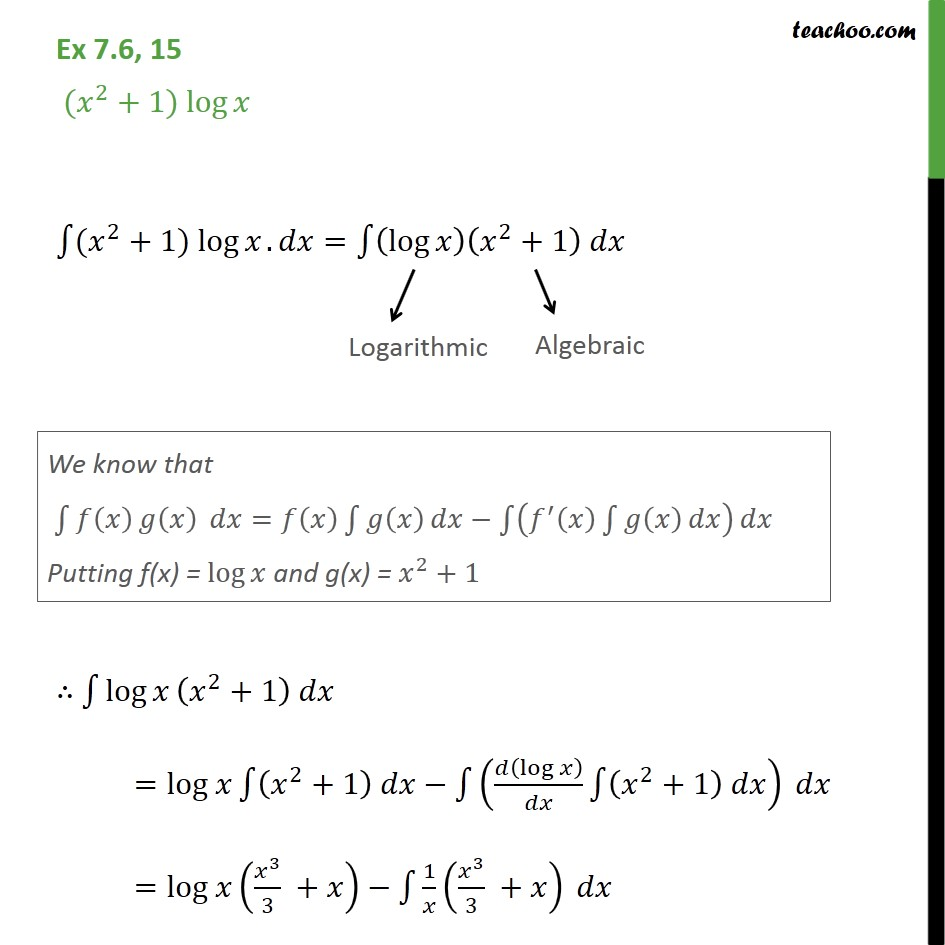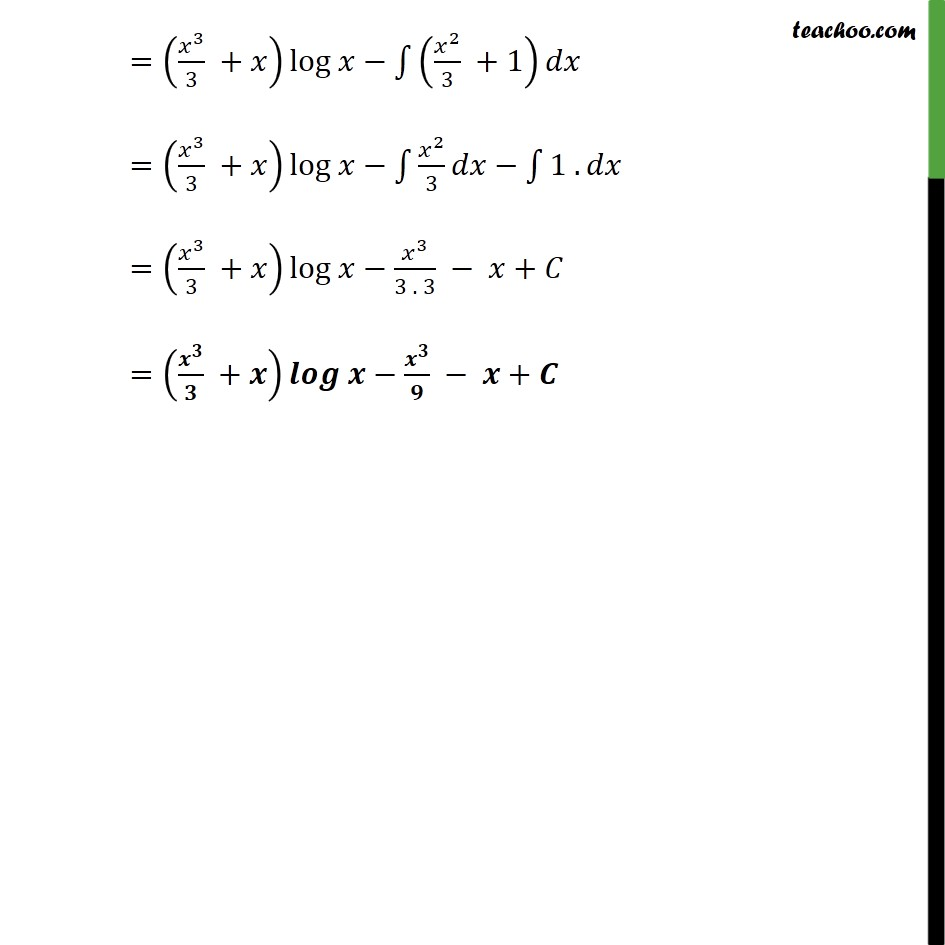1. Chapter 7 Class 12 Integrals (Term 2)
2. Serial order wise
3. Ex 7.6

Transcript

Ex 7.6, 15 ( 2 +1) log ( 2 +1) log . = log 2 +1 log 2 +1 = log 2 +1 log 2 +1 = log 3 3 + 1 3 3 + = 3 3 + log 2 3 +1 = 3 3 + log 2 3 1 . = 3 3 + log 3 3 . 3 + = + +

Ex 7.6

Chapter 7 Class 12 Integrals (Term 2)
Serial order wise